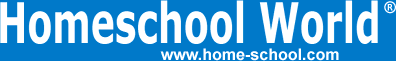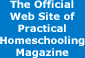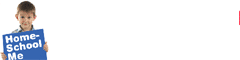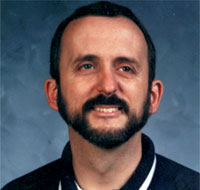Home Curriculum Catalog Articles Contests Events Groups Forum News ContactGetting Started Preschool/K Elementary Middle School High School College & Career Prep Special Needs Group Activities Research FAQs# Primes, Part II: Using Higher Math to Reinforce and Practice Arithmetic

By Bob Hazen
Printed in Practical Homeschooling #32, 1999.What you never knew about the Sieve of Eratosthenes. Division practice with a difference!Recently I introduced the idea of using higher math as a means of reinforcing and practicing basic arithmetic facts. The novelty and curiosity of higher math concepts can allow basic facts to be practiced in new ways in which children see some of the "cool" things about mathematics, such as its high degree of orderliness and its power to predict. When parents overuse the traditional tools of drill sheets and flashcards, it's often for lack of better methods. These columns on "using higher math" offer some alternatives.

A Review of Prime Numbers

A previous column dealt with one of my favorite activities with higher math concepts: investigating prime numbers. Remember, a prime number is a whole number that is divisible only by two different whole numbers. Thus, 2, 7, and 11 are prime numbers because they can be divided by exactly and only two different numbers: 1 and the number itself. The numbers 6, 15, and 27 are not prime because in addition to being divisible by themselves and 1, they each are also divisible by 3. The number 49 can be divided by 1 and 49 - but it can also be divided by 7, so 49 is not prime. Numbers that are divisible by more than 2 whole numbers are called "composite," so 6, 15, 27, and 49 are composite.

The prime numbers less than 200 are 2, 3, 5, 7, 11, 13, 17, 19, 23, 29, 31, 37, 41, 43, 47, 53, 59, 61, 67, 71, 73, 79, 83, 89, 97, 101, 103, 107, 109, 113, 127, 131, 137, 139, 149, 151, 157, 163, 167, 173, 179, 181, 191, 193, 197, and 199. Refer to this list for later use in the activities described below.

Note three characteristics of prime numbers. First, the only even prime number is 2. Every even number greater than 2 is a composite number. Second, every prime number greater than 2 is an odd number (3, 5, 7, 11, 13, etc.), although not every odd number is prime (9, 15, and 21 are not prime). Third, interestingly enough, the number 1 is not considered prime, simply because it has exactly one divisor - the number 1. But 1 is also not considered composite - because composite numbers have 3 or more divisors. So because 1 uniquely has exactly one divisor, we simply put 1 off in a corner by itself, neither prime nor composite.

Primes & Division Practice

Prime numbers can be used for children who need to practice the long-division procedure. Instead of practicing long division with a page of 20-30 exercises from your math book, occasionally have your child use long division to discover or become familiar with prime numbers.

Using the list of prime numbers from earlier in this article, pick two prime numbers (e.g., 59 and 73) along with two odd composite numbers that are not on the list (e.g., 49 and 91) - but do not let your child know which is which. Then show your child those four numbers: 49, 59, 73, and 91, and say, "You only have four numbers to divide today! Your assignment is to use division to find out which of these four numbers is prime and which is composite."

For the lowest number of 49, your child will have to try dividing by 2, then 3, then 4, and so on. (See if your child can figure out at which number you no longer need to divide.) If 49 is composite, then it will be divisible by either 2 or by 3 or by another higher number. Remember that "divisible by" means having a remainder of zero when division is completed. For these four numbers, most long-division novices will have to do as much practice with this division procedure as they do for an entire page of textbook division exercises.

This type of "replacement" practice can be done anytime your math text assigns a block of long-division exercises (which usually is quite often). Occasionally replace the textbook division exercises with the assignment to use division practice to determine which of a handful of numbers are prime and which are composite.

You can also extend this to higher numbers in the hundreds for older students who need more challenging division practice. Simply pick some odd whole numbers and go from there - "Your assignment today is to use division on 127, 131, 143, and 221 to find out which numbers are prime and which are composite - and the only calculator you can use is the one in your head."

A variation on this use of prime numbers with division is a multi-day project. Instead of providing a short list of numbers that you select, have your child use the long-division procedure to examine (over several days) all the odd numbers up to, for example, 100. On the first day, depending on ability and number sense, your child might do long division on the odd numbers 11, 13, 15, 17, and 19, finding that 15 is the only one that is not prime. The next day's session might only examine 21, 23, 25, 27, and 29, finding that 21, 25, and 27 are not prime. The third session might use long division to examine the odd numbers in the 30's - 31, 33, 35, 37, and 39 - to find which ones are prime. Again, work with higher numbers for more advanced students - have them find all the prime numbers between 200 and 300, or between 400 and 500.

Remember that more than one long-division procedure will be done for each number that is being checked. Solely for the number 21, a child may have to divide by both 2 and 3 to find out that 21/3 = 7, which means that 21 is not prime. Likewise, just for the number 23, a child may have to write out numerous procedures (dividing by 2, 3, 4, 5, 6, 7, 8, etc.) before discovering that dividing any number (except for 1 and 23) into 23 always leaves a remainder, thus confirming that 23 is prime.

Filtering Out the Prime Numbers

A classic and formal way to investigate and organize prime numbers is the procedure called the Sieve of Eratosthenes. Like all sieves, this filtering device is meant to strain out certain items (the composite numbers) while letting other items (the prime numbers) pass through. This procedure is explained in Chart 1 on page 34.

This activity with a short list of the Sieve of Eratosthenes can be done by students who are just beginning the long division procedure (usually in fourth grade). Older students can work with a longer list of numbers - up to 100 or even 200. Similar extended work can be done with longer lists for older students working with the division exercises mentioned above.

Summary

Note well that connecting higher math with basic arithmetic produces benefits in both directions. In the arithmetic direction, activities with the higher math topic of prime numbers reinforce and support the practice of basic arithmetic facts, providing one more avenue for the practice necessary to lead to basic fact mastery. In the higher math direction, practicing arithmetic with a higher math topic like prime numbers provides a preview of some advanced - and interesting! - topics that are waiting down the mathematical road.

The practice of arithmetic facts can be something that it wasn't for too many of us adults - it can be intriguing and fun. The wise teacher uses curiosity and novelty to increase learning. Prime numbers are one way to use novelty and curiosity to practice and reinforce basic arithmetic facts. Mathematicians have long beheld prime numbers with a mixture of fascination and wonder. Let your children begin to see some of that fascination themselves.Terms of Use   Privacy Policy Copyright ©1993-2023 Home Life, Inc.